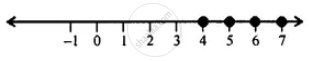# Solve and Graph the Solution Set on a Number Line: 8x – 9 > 35 – 3x ; X ∈ N - Mathematics

Sum

Solve and graph the solution set on a number line: 8x – 9 > 35 – 3x ; x ∈ N

#### Solution

8x – 9 ≥ 35 – 3x

⇒ 8x + 3x - 9 ≥ 35 - 3x + 3x   ...(Adding 3x to both sides)

⇒ 11x - 9 ≥ 35

⇒ 11x - 9 + 9 ≥ 35 + 9  ..(Adding 9 to both sides)

⇒ 11x ≥ 44

⇒ "11x"/11 ≥ 44/11  ...(Dividing both side by 11)

⇒ x ≥ 4

∴ The required graph is:Concept: Operation on of Whole Number on Number Line
Is there an error in this question or solution?

#### APPEARS IN

Selina Concise Mathematics Class 8 ICSE
Chapter 15 Linear Inequations
Exercise 15 (B) | Q 6 | Page 175